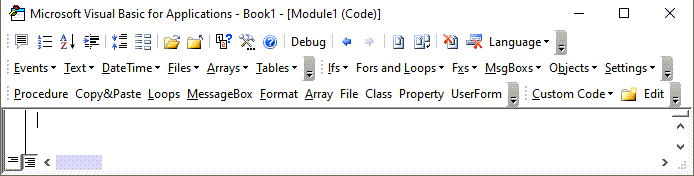# VBA CInt Function – Convert to Integer

This tutorial will demonstrate how to use the CInt VBA function to convert an expression to the integer data type.

## CInt Function

### VBA CInt Convert Expression to Integer

The VBA CInt function can be used to convert expressions to integer data type inside VBA code. The resulting number is rounded to become an integer.

``````Sub CIntExample_1()
MsgBox CInt(12.34)  'Result is: 12
MsgBox CInt(12.345) 'Result is: 12
MsgBox CInt(-124)   'Result is: -124
MsgBox CInt(-12.34) 'Result is: -12
End Sub
``````

### VBA CInt Rounding

The VBA CInt function will round the decimal part of a number type or a number like expression. However, it does not round correctly in all cases. When the decimal part is 0.5 then VBA CInt function returns the closest even integer.

``````Sub CIntExample_2()
MsgBox CInt(0.34)
'Result is:    0

MsgBox CInt(0.99)
'Result is:    1

MsgBox CInt(-124.95)
'Result is:    -125

MsgBox CInt(1.5)
'Result is:    2

MsgBox CInt(2.5)
'Result is:    2
End Sub
``````

We can add a decimal number relatively small to our expected decimal value to change the behavior of VBA Cint function to the expected.

``````Sub CIntExample_3()
MsgBox CInt(2.5)
'Result is:    2
MsgBox CInt(2.5 + 0.001)
'Result is:    3

MsgBox CInt(14.5)
'Result is:   14
MsgBox CInt(14.5 + 0.001)
'Result is:   15
End Sub
``````

### VBA CInt Converting Strings to Integers

The VBA CInt function can be used to convert strings to integers if the characters in the string have a meaning as numbers.

``````Sub CIntExample_4()
Dim StrEx As String
StrEx = "112"
MsgBox CInt(StrEx)
'Result is: 112

StrEx = "112.3"
MsgBox CInt(StrEx)
'Result is: 112   --> 112.3 is rounded

StrEx = "11,2"
MsgBox CInt(StrEx)
'Result is: 112   -->  , is ignored

StrEx = "\$112"
MsgBox CInt(StrEx)
'Result is: 112   -->  \$ is ignored
End Sub
``````

### VBA CInt Run-Time Error 13 Type Mismatch

Using VBA Cint function with strings that contain non-numerical characters or characters that don’t have meaning in numerical context will result in a Run-Time error ’13’: Type mismatch.

``````Sub CIntExample_5()
'The code below will result in an ERROR message
'CInt can’t handle non numerical characters
Dim StrEx As String
StrEx = "Ab13"
MsgBox CInt(StrEx)
End Sub
``````

### VBA CInt Run-Time Error 6 Overflow

Using VBA Cint function with strings that result in a value smaller or bigger than the expected integer will result in a Run-Time error ’6’: Overflow. Integer data type in excel has an expected value of -32768 to 32767.

``````Sub CIntExample_6()
'The code below will result in an ERROR message
'CInt cant handle non numerical characters
Dim StrEx As String
StrEx = "1234567"
MsgBox CInt(StrEx)
End Sub
``````

Stop searching for VBA code online. Learn more about AutoMacro - A VBA Code Builder that allows beginners to code procedures from scratch with minimal coding knowledge and with many time-saving features for all users!### VBA CInt Regional Settings

VBA CInt function has different behavior converting strings with comma or dot.  It uses the Regional Settings of the operating system for decimal separator and digit separator.

``````Sub CIntExample_7()
Dim StrEx As String
StrEx = "1,9"
MsgBox CInt(StrEx)
‘If Regional settings have , as a grouping separator then
'Result is: 19
‘If Regional settings have , as a decimal separator then
'Result is: 2 (2 because 1.9 gets rounded)

StrEx = "1.9"
MsgBox CInt(StrEx)
‘If Regional settings have . as a grouping separator then
'Result is: 19
‘If Regional settings have . as a decimal separator then
'Result is: 2 (2 because 1.9 gets rounded)
End Sub
``````

### VBA CInt Converting Booleans to Integers

VBA Cint function can convert boolean variables to integers. If the evaluated expression is true the resulting integer is -1 and if the evaluated expression is false, the resulting integer is 0.

``````Sub CIntExample_8()
Dim BoolEx As Boolean
BoolEx = True
MsgBox CInt(BoolEx)  'Result is: -1
MsgBox CInt(2 = 2)   'Result is: -1

BoolEx = False
MsgBox CInt(BoolEx)  'Result is: 0
MsgBox CInt(1 = 2)   'Result is: 0
End Sub
``````

### VBA CInt Converting Dates to Integers

VBA Cint function can convert a date variable to an integer. The returned value is the internal number used by excel for date storage rounded. If that number is outside of the expected integer limits for VBA then we get a  Run-Time error ’6’: Overflow.

``````Sub CIntExample_9()
Dim DateEx As Date
DateEx = #2/3/1940#
MsgBox CInt(DateEx)
'Result is: 14644
DateEx = #8/7/1964#
MsgBox CInt(DateEx)
'Result is: 23596
End Sub
``````